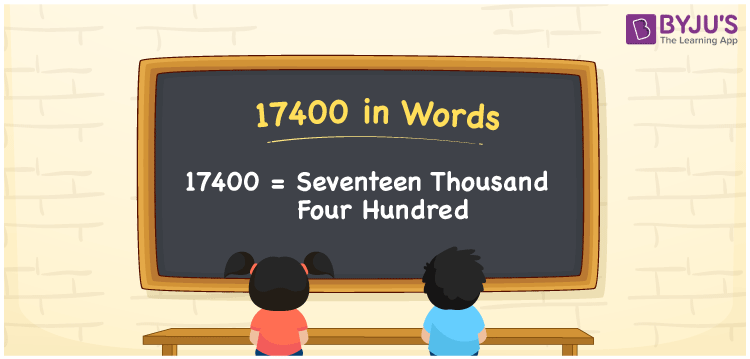# 17400 in Words

We can write 17400 in words as Seventeen thousand four hundred. Suppose you bought a new bicycle that costs Rs. 17400, then you can say, “I bought a new bicycle worth Seventeen thousand four hundred rupees”. In this article, you will learn how to write the cardinal number 17400 in words with the help of a place value chart and some interesting facts about 17400.

 17400 in words Seventeen thousand four hundred Seventeen thousand four hundred in Numbers 17400

## 17400 in English Words

Generally, we use the English alphabet to write numbers in words. Thus, we can spell 17400 in English words as “Seventeen thousand four hundred”.## How to Write 17400 in Words?

As we know, 17400 is a five-digit number. Thus, we need to make a five-column place value chart to convert 17400 into words, as shown below.

 Ten thousand Thousands Hundreds Tens Ones 1 7 4 0 0

Here, ones = 0, tens = 0, hundreds = 4, thousands = 7, ten thousand = 1

By expanding these digits based on the place values, we get;

1 × Ten thousand + 7 × Thousand + 4 × Hundred + 0 × Ten + 0 × One

= 1 × 10000 + 7 × 1000 + 4 × 100 + 0 × 10 + 0 × 1

= 10000 + 7000 + 400

= Ten Thousand + Seven thousand + Four hundred

= Seventeen thousand + Four hundred

= Seventeen thousand four hundred

17400 is a natural number that is the successor of 17399 and predecessor of 17401.

17400 in words – Seventeen thousand four hundred

Is 17400 an odd number? – No

Is 17400 an even number? – Yes

Is 17400 a prime number? – No

Is 17400 a composite number? – Yes

Is 17400 a perfect square number? – No

Is 17400 a perfect cube number? – No

## Frequently Asked Questions on 17400 in Words

Q1

### How do you write 17400 in words?

Using English words, we spell 17400 as Seventeen thousand four hundred.
Q2

### How do you mention the amount Rs. 17400 in words?

We can mention the amount of Rs. 17400 in words as Seventeen thousand four hundred rupees.
Q3

### What is the number name of 17400?

The number name of 17400 is Seventeen thousand four hundred.